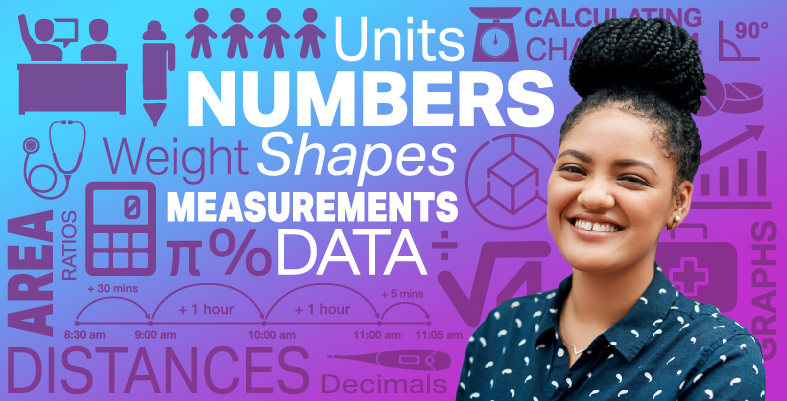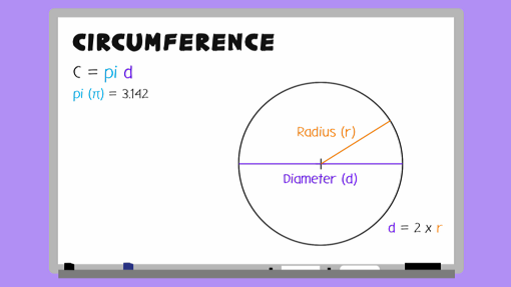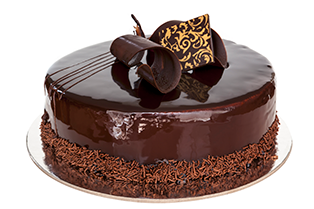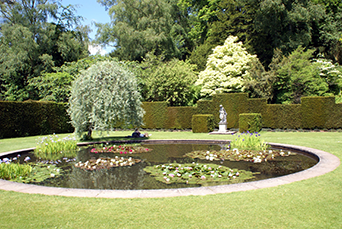Everyday maths for Health and Social Care and Education Support 2

Start this free course now. Just create an account and sign in. Enrol and complete the course for a free statement of participation or digital badge if available.

Free course

# 1.3 Circumference of a circle

You may have noticed that a new term has slipped in to the title of this section. The term circumference refers to the distance around the outside of a circle – its perimeter. The perimeter and the circumference of a circle mean exactly the same, it’s just that when referring to circles you would normally use the term circumference.Skip transcript

#### Transcript

The circumference of a circle is the distance around its edge. That is, its perimeter. Before learning how to work out the circumference of a circle, look at these two key terms: diameter and radius. Radius, or r, is the distance from the centre of the circle to the edge. Diameter, or d, is the distance from one edge of the circle to the other, passing through the centre of the circle.

You can see the diameter is always double the radius. If you know either the radius or the diameter, you can always work out the other. And you can also work out the circumference. Here is the basic formula you need to work out the circumference of a circle: Circumference = pi x diameter. This can also be written as C = pi x d, or pi d.

Pi, or this symbol (which is the Greek letter used to represent it), is a constant number that is around the value of 3.142. It's a number that goes on for ever, so you tend to shorten it to the more manageable number of 3.14 or 3.142. In technical terms, pi is the number you get if you divide a circle's circumference by its diameter, and it's the same for every circle.

Let's look at an example of calculating circumference. This circle has a diameter of 5 centimetres. You can put this into the formula, so you get C = pi x 5. Look for the pi key on your calculator, or you can use the shortened version, 3.142. So the circumference, C = 3.142 x 5, which equals 15.71 centimetres.

Here's another example, but this time the radius is labelled. How would you calculate the circumference? Remember that the formula for circumference uses diameter, so you'll need to work this out first. Since the radius is 12 centimetres, the diameter will be double this. 12 x 2 = 24 centimetres, so d equals 24 centimetres. Now using the formula, circumference = 3.142 x 24, which equals 75.408.

Now, try the examples in the next activity.

End transcript

Interactive feature not available in single page view (see it in standard view).

## Activity 3: Finding the circumference

1. You have made a cake to celebrate a residents 95th birthday.

The diameter of the cake is 15 cm. You have a length of ribbon that is 0.5 m long. Will you have enough ribbon to go around the outside of the cake?Figure 11 A round chocolate cake
2. The nursery has just installed a pond in its outside space and needs to put a fence around it for safety. The radius of the pond is 7.4 m.

What length of fencing would you require to fit around the perimeter of the pond? Round your answer up to the next full metre.Figure 12 A round garden pond

1. d = 15 cm

Using the formula C = πd

•       C = 3.142 × 15

•       C = 47.13 cm

Since you need 47.13 cm and have ribbon that is 0.5 m (50 cm) long, yes, you have enough ribbon to go around the cake.

2. C = πd

C = 3.142 × 14.8

C = 46.5016 m which is 47 m to the next full metre.

You should now be feeling confident with finding the perimeter of all types of shapes, including circles. By completing Activity 3, you have also re-capped on using formulas and rounding.

The next part of this section looks at finding the area (space inside) a shape or space. As mentioned previously, this is incredibly useful in everyday situations such as working out how much carpet or turf to buy, how many rolls of wallpaper you need or how many tins of paint you need to give the wall two coats.

## Summary

In this section you have learned:

• that perimeter is the distance around the outside of a space or shape

• how to find the perimeter of simple and more complex shapes

• how to use the formula for finding the circumference of a circle.

FSM_SSH_2